## A person holding a lunch bag is moving upward in a hot air balloon at a constant speed of 7.7 m/s . When the balloon is 21 m above the groun

Question

A person holding a lunch bag is moving upward in a hot air balloon at a constant speed of 7.7 m/s . When the balloon is 21 m above the ground, she accidentally releases the bag. Part A What is the speed of the bag just before it reaches the ground

in progress 0
6 months 2021-07-29T10:00:26+00:00 1 Answers 46 views 0

The speed of the bag just before it reaches the ground is 21.71 m/s.

Explanation:

The speed of the bag can be found using the following equation: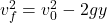Where: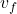is the final speed =?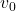is the initial speed = 7.7 m/s

g: is the gravity = 9.81 m/s²

y: is the height = 21 m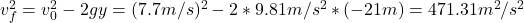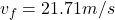Therefore, the speed of the bag just before it reaches the ground is 21.71 m/s.

I hope it helps you!